# Power Grid Network

An undirected, unweighted network representing topology

An undirected, unweighted network representing the topology of the Western States Power Grid of the United States.

(4941 vertices, 6594 edges)

## Examples

### Basic Examples

Retrieve the graph:

 In:=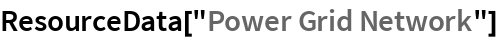Out=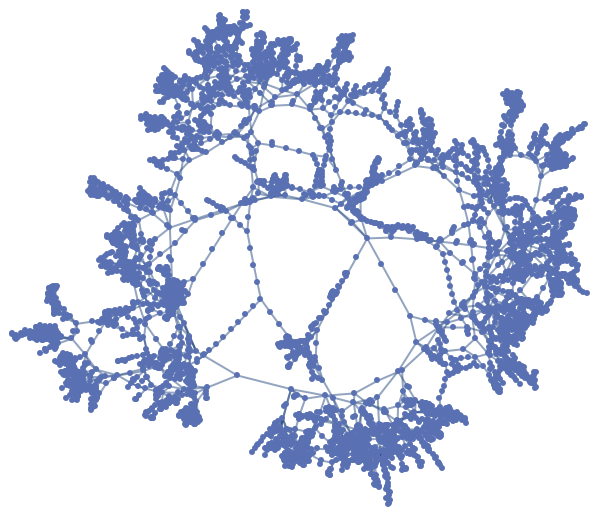Summary properties:

 In:=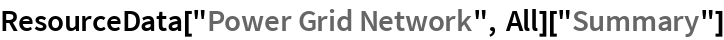Out=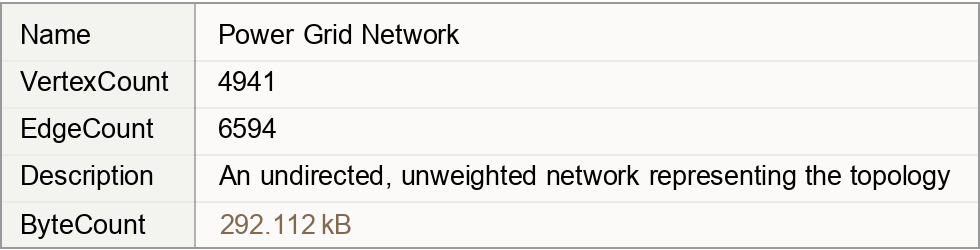### Basic Applications

Show the most connected vertex:

 In:=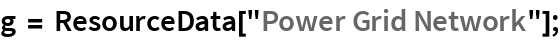In:=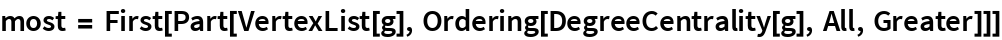Out=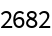In:=Out=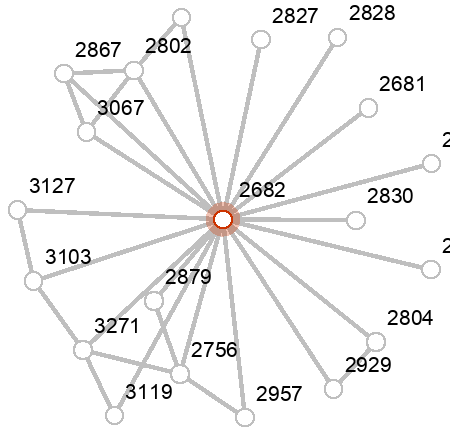Wolfram Research, "Power Grid Network" from the Wolfram Data Repository (2019)